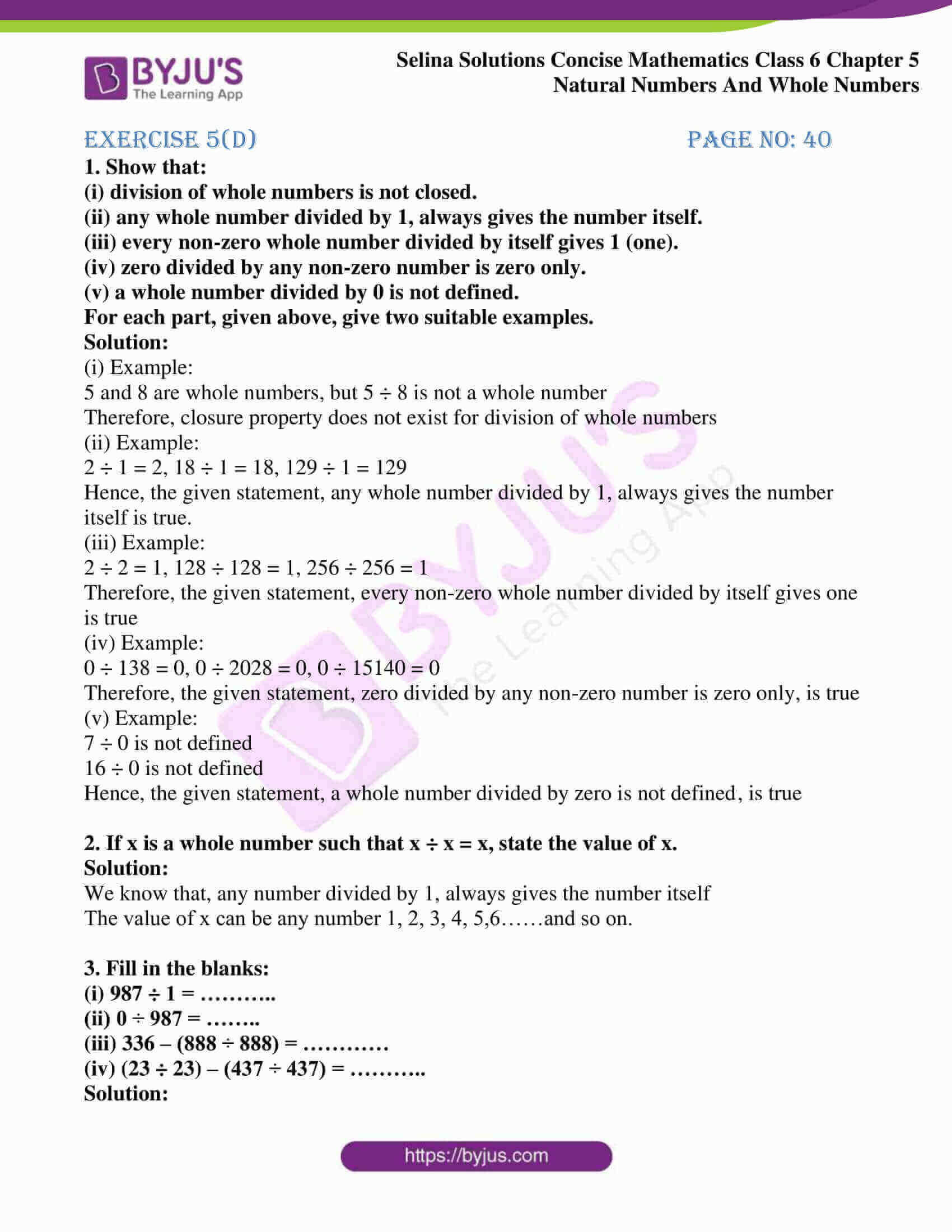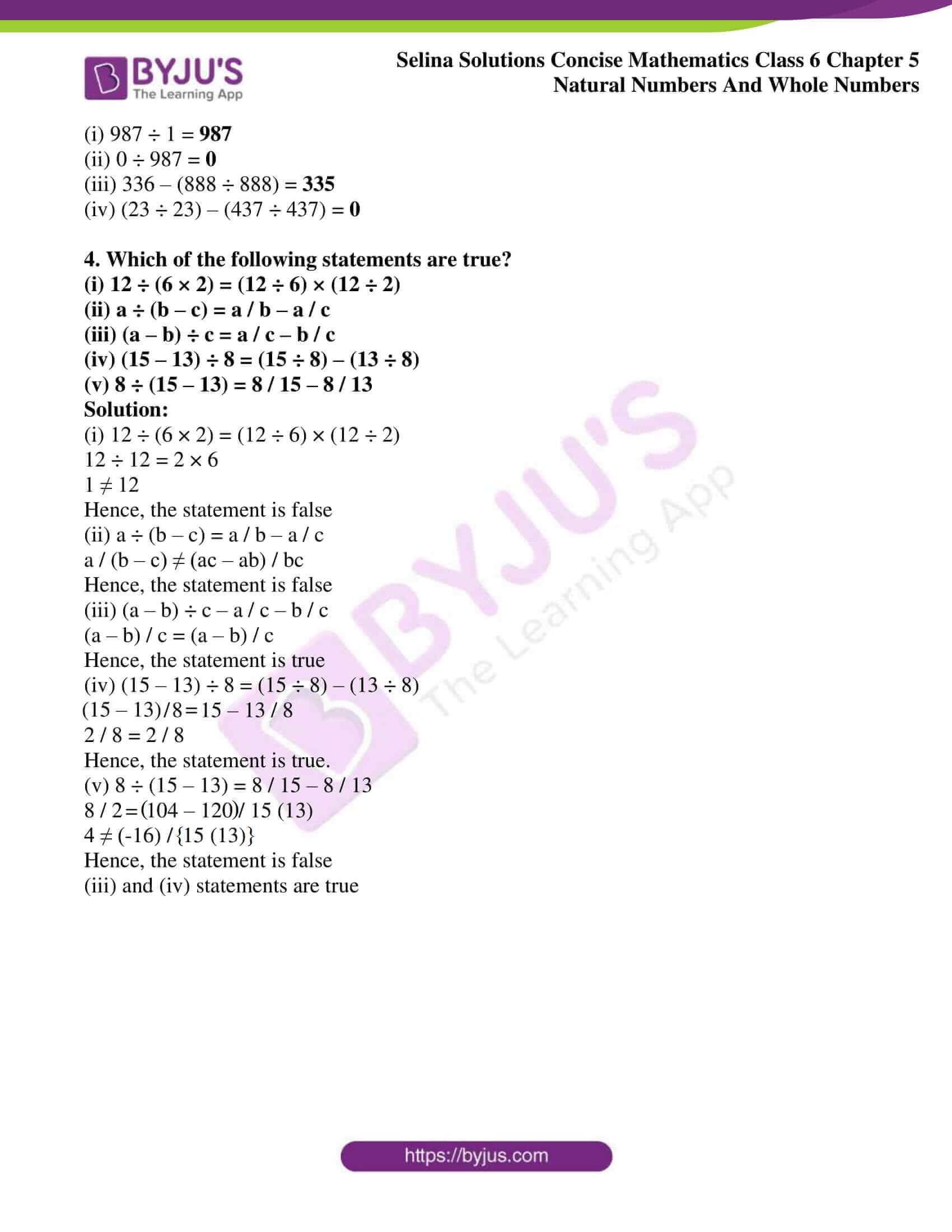# Selina Solutions Concise Mathematics Class 6 Chapter 5: Natural And Whole Numbers Exercise 5(D)

Selina Solutions Concise Mathematics Class 6 Chapter 5 Natural Numbers And Whole Numbers Exercise 5(D) provide problems on division of Whole Numbers for better conceptual knowledge among students. The solutions are created by experts at BYJU’S, in accordance with the students’ understanding skills. Practicing these Solutions enables students to solve the textbook questions in a short duration without any errors. These solutions provide step by step methods in a simple and lucid manner to enhance their problem-solving abilities. Students can access Selina Solutions Concise Mathematics Class 6 Chapter 5 Natural Numbers And Whole Numbers Exercise 5(D) PDF, for free download from the links provided below.

## Selina Solutions Concise Mathematics Class 6 Chapter 5: Natural Numbers And Whole Numbers Exercise 5(D) Download PDF### Access other exercises of Selina Solutions Concise Mathematics Class 6 Chapter 5: Natural Numbers And Whole Numbers

Exercise 5(A) Solutions

Exercise 5(B) Solutions

Exercise 5(C) Solutions

Exercise 5(E) Solutions

Exercise 5(F) Solutions

### Access Selina Solutions Concise Mathematics Class 6 Chapter 5: Natural Numbers And Whole Numbers Exercise 5(D)

#### Exercise 5(D) page no: 40

1. Show that:

(i) division of whole numbers is not closed.

(ii) any whole number divided by 1, always gives the number itself.

(iii) every non-zero whole number divided by itself gives 1 (one).

(iv) zero divided by any non-zero number is zero only.

(v) a whole number divided by 0 is not defined.

For each part, given above, give two suitable examples.

Solution:

(i) Example:

5 and 8 are whole numbers, but 5 ÷ 8 is not a whole number

Therefore, closure property does not exist for division of whole numbers

(ii) Example:

2 ÷ 1 = 2, 18 ÷ 1 = 18, 129 ÷ 1 = 129

Hence, the given statement, any whole number divided by 1, always gives the number itself is true.

(iii) Example:

2 ÷ 2 = 1, 128 ÷ 128 = 1, 256 ÷ 256 = 1

Therefore, the given statement, every non-zero whole number divided by itself gives one is true

(iv) Example:

0 ÷ 138 = 0, 0 ÷ 2028 = 0, 0 ÷ 15140 = 0

Therefore, the given statement, zero divided by any non-zero number is zero only, is true

(v) Example:

7 ÷ 0 is not defined

16 ÷ 0 is not defined

Hence, the given statement, a whole number divided by zero is not defined, is true

2. If x is a whole number such that x ÷ x = x, state the value of x.

Solution:

We know that, any number divided by 1, always gives the number itself

The value of x can be any number 1, 2, 3, 4, 5,6……and so on.

3. Fill in the blanks:

(i) 987 ÷ 1 = ………..

(ii) 0 ÷ 987 = ……..

(iii) 336 – (888 ÷ 888) = …………

(iv) (23 ÷ 23) – (437 ÷ 437) = ………..

Solution:

(i) 987 ÷ 1 = 987

(ii) 0 ÷ 987 = 0

(iii) 336 – (888 ÷ 888) = 335

(iv) (23 ÷ 23) – (437 ÷ 437) = 0

4. Which of the following statements are true?

(i) 12 ÷ (6 × 2) = (12 ÷ 6) × (12 ÷ 2)

(ii) a ÷ (b – c) = a / b – a / c

(iii) (a – b) ÷ c = a / c – b / c

(iv) (15 – 13) ÷ 8 = (15 ÷ 8) – (13 ÷ 8)

(v) 8 ÷ (15 – 13) = 8 / 15 – 8 / 13

Solution:

(i) 12 ÷ (6 × 2) = (12 ÷ 6) × (12 ÷ 2)

12 ÷ 12 = 2 × 6

1 ≠ 12

Hence, the statement is false

(ii) a ÷ (b – c) = a / b – a / c

a / (b – c) ≠ (ac – ab) / bc

Hence, the statement is false

(iii) (a – b) ÷ c – a / c – b / c

(a – b) / c = (a – b) / c

Hence, the statement is true

(iv) (15 – 13) ÷ 8 = (15 ÷ 8) – (13 ÷ 8)

(15 – 13) / 8 = 15 – 13 / 8

2 / 8 = 2 / 8

Hence, the statement is true.

(v) 8 ÷ (15 – 13) = 8 / 15 – 8 / 13

8 / 2 = (104 – 120) / 15 (13)

4 ≠ (-16) / {15 (13)}

Hence, the statement is false

(iii) and (iv) statements are true